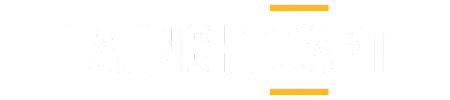# Programming a Driverless Car Chapter 5: Linear Regression

Contents

• Working with a regression Problem
• Defining regression Equation
• Cost Function
• Parameters of cost function
• Objective of Linear Regression
• Different Regression Methods

Let’s understand this with an example. We have 2 types of data : Input data and output data.So, we have a table of data with us. We can portray the same data on a graph. So,graph can be made between price and area. Both the axis are:
Y- Dependent Variable, criterion variable or regressand
X- Independent Variable, predictor variables or regressors.
There is no connection between the data, by which we can predict values. The data is all scattered, so to predict a connection between any 2 values, we draw a straight line between the points. The straight line is a model used to create a value for an independent input so we can get a dependent output.We have chosen points that are very far away from the current model but it passes it from few points. You can call this prior knowledge. Now, how accurate the predicted values will be depends on how optimized the model is.So, if we provide more data, it can be more optimized and give better predictions. The current model is a straight line and the equation for it will be:
y=mx+c —– Linear regression in one variable
As input we have 2 types of value, X(independent variable) and Y (Dependent Variable)
If we had 2 variables then it would have been multiple variable linear regression.
Solving equations for the above model. The straight line equation is:
y=mx+c
We can change x and c to w(0) and w(1):The main goal for linear regression is minimizing the difference between y and y. If we predict new values for each data set, so it make our current equation more optimized. But how do we minimize the difference?
When we look at various examples, we tend to see that how various intercept value changes based on the value of m and c.The Cost Function for this will be:Value of i is taken from 1 to m, to train the model for different models. y-yvalue has to be decreased to generate a more optimum solution. Therefore, we have squared the value of its difference.
Objective of Linear Regression:

• Establish if there is a relationship between two variables. Example- relationship between housing process and area of house, no of hours of study and the marks obtained, income and spending etc.
• Prediction of new possible values. Based on the area of house predicting the house prices in a particular month, based on number of hour studied predicting the possible marks. Sales in next 3 months etc.

Regression Algorithms:

• Simple Linear Regression
Where we take 1 dependent variable (interval or ratio), 1 independent variable (interval or ratio or dichotomous)
• Multiple Linear Regression
Where we have 1 dependent variable (interval or ratio), 2+ independent variable (interval or ratio or dichotomous)
• Logistic regression
1 dependent variable (binary), 2+ independent variable(s) (interval or ratio or dichotomous)

• What is GDA?
• Learning rate
• Overview

The goal is to optimize Y, so it can achieve the value of the dependent variable Y.Where X is the independent variable and Y is the value predicted by the current model.
Repeat this until convergence:While updating w=0, w=1
:= is an assignment operator.
is learning rate.
Learning rate controls how big step we take while updating our parameter w.
If is too small, gradient descent can be slow.
If is too big, gradient descent can overshoot the minimum, it may fail to converge.LAB: Linear Regression Example using sklearn

`>>>import numpy >>>import matplotlib`
`>>>import sklearn `
`import matplotlib.pyplot as plt import numpy as np`
from sklearn import datasets, linear_model

`diabetes=datasets.load_diabetes()`
`diabetes_x=diabetes.data[:,np.newaxis,2]`
#for testing and training
`diabetes_x_train=diabetes_x[:-30]`
`diabetes_x_testing=diabetes_x[-30:]`
#for training
`diabetes_y_train=diabetes.target[:-30]`
`diabetes_y_testing=diabetes.target[-30:]`
#creating model using linear model
`reg=linear_model.Linearregression()`
`reg.fit(diabetes_x_train,diabetes_y_train)`
`plt.scatter(diabetes_x_testing,diabetes_y_testing,color= ‘red’) ----this is for testing in a graph form`
`plt.plot(diabetes_x_testing,reg.predict(diabetes_x_testing),color= ‘green’,linewidth=3)`
`plt.show()`
Output:Fig:Output of the program
Here we see, the testing data is scattered and the training model is predicted using linear regression model. This is the most optimized output.
LAB2: Using Linear regression using Gradient descent Algorithm
Working with X=independent Variable , Y=Dependent VariableWe have these 2 data sets, where the first one is for X and the other one is Y.
This is our data:Code:
`import theano`
`import numpy`
`import matplotlib.pyplot as plt`
#getting both independent and dependent data values
`X=numpy.asarray([3,4,5,6.1,6.3,2.88,8.89,5.62,6.9,1.97,8.22,9.81,4.83,7.27,5.14,3.08])`
`Y=numpy.asarray([0.9,1.6,1.9,2.9,1.54,1.43,3.06,2.36,2.3,1.11,2.57,3.15,1])`
#we will feed these values in regression model
`m=numpy.random.randn()`
`c=numpy.random.randn()`
`ms=theano.shared(m,name= ‘ms’)`
`cs=theano.shared(c,name= ‘cs’)`
`x=theano.tensor.vector( ‘x’)`
`y=theano.tensor.vector( ‘y’)`
`yh=theano.tensor.dot(x,ms)+cs`
`n=X.shape`
`cost=theano.tensor.sum(theano.tensor.pow(yh-y,2))/(2*n)`
`gradient= theano.tensor.grad(cost,mss)`
`gradc=theano.tensor.grad(cost,c)`
`alpha=0.01`
`steps=10000`
`mn=ms-alpha*gradm`
`cn=cs-alpha*gradc`
`train=theano.function([x,y,,cost,updates=[(ms,mn),(cs,cn)]])`
`test=theano.function([x],yh)`
For i in range(steps):
`costm=train(X,Y)`
`print costm`
`print ‘intercept’`
`print cs.get_value()`
`print ‘slope’`
`print ms.get_value()`
#for testing the data
`a=numpy.linspace(0,10,10) b=test(a) `
`plt=scatter(X,Y)`
`plt.plot(a,b)`
`plt.show()`
Output: This is the output if we have to train it.Output: This is the data for testing data values.Best mean Fitting-
In this we will see how do we use Linear regression to predict better values.

• Best Fitting Line
• Calculating Slope
• Calculating Intercept

Best fitting line-
A line that fits the data “best” will be one for which the n prediction errors-one for each observed data point- are as small as possible in some overall sense.
In linear regression we establish a relationship between x and y. Like this, what we have already seen
For calculating slope:Instead of using gradient descent algorithm we will use best fitting line`import numpy`
`import matplotlib.pyplot as plt`
`x=numpy.asarray([1,2,3,4,5,6])`
`y=numpy.asarray([5,4,6,7,5,6])`
`plt.scatter(x,y)`
`plt.show()`
This is the data set:`import numpy`
`import matplotlib.pyplot as plt`
`x=numpy.asarray([1,2,3,4,5,6])`
`y=numpy.asarray([5,4,6,7,5,6])`
`def bestfit(x,y):`
` m=((numpy.mean(x)*numpy.mean(y))-(numpy.mean(x*y)))/(numpy.mean(x)**2-(numpy.mean(x*x))`
`b=(numpy.mean(y))-(m*numpy.mean(x))`
`return m,b`
`m,b=bestfit(xd,yd)`
`yh=[]`
`For x in xd:`
`yh.append(m*x+b)`
`print m`
`print b`
`plt.scatter(x,y)`
`plt.show()`

Recommended Reading:  Introduction to Supervised learning
Next: Chapter 6: Clustering